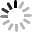Events

# Aixton Classroom : 10 basic knowledge of fiber optic cable

August 25, 20221. Briefly describe the composition of optical fibers?

A: An optical fiber consists of two basic parts: the core and cladding made of transparent optical material, and the coating.

2. What are the basic parameters that describe the transmission characteristics of optical fiber lines?

A: Including loss, dispersion, bandwidth, cutoff wavelength, mode field diameter, etc.

3. What is the cause of fiber attenuation?

Answer: The attenuation of a fiber refers to the reduction in optical power between two cross-sections of a fiber, which is related to the wavelength. The main causes of attenuation are scattering, absorption, and optical losses due to connectors and splices.

4. How is the fiber attenuation coefficient defined?

Answer: Defined by the attenuation (dB/km) per unit length of a uniform fiber in steady state.

5. What is insertion loss?

Answer: It refers to the attenuation caused by inserting optical components (such as inserting connectors or couplers) in the optical transmission line.

6. What is the bandwidth of optical fiber related to?

Answer: The bandwidth of the fiber refers to the modulation frequency when the amplitude of the optical power is 50% or 3dB lower than the amplitude of the zero frequency in the transfer function of the fiber. The bandwidth of an optical fiber is approximately inversely proportional to its length, and the product of the bandwidth length is a constant.

7. How many kinds of dispersion are there in optical fiber? What is it about?

Answer: The dispersion of an optical fiber refers to the expansion of the group delay in a fiber, including modal dispersion, material dispersion and structural dispersion. Depends on the characteristics of both the light source and the fiber.

8. How to describe the dispersion characteristics of the signal propagating in the fiber?

Answer: It can be described by three physical quantities, pulse broadening, fiber bandwidth, and fiber dispersion coefficient.

9. What is the cutoff wavelength?

Answer: It refers to the shortest wavelength that can only transmit the fundamental mode in the fiber. For single-mode fibers, the cutoff wavelength must be shorter than the wavelength of the transmitted light.

10. What effect will the dispersion of optical fiber have on the performance of optical fiber communication system?

Answer: The dispersion of the fiber will cause the optical pulse to broaden during the transmission in the fiber. It affects the size of the bit error rate, the length of the transmission distance, and the size of the system rate.

The phenomenon of optical pulse broadening in optical fibers caused by different group velocities of different wavelengths in the spectral composition of the light source.